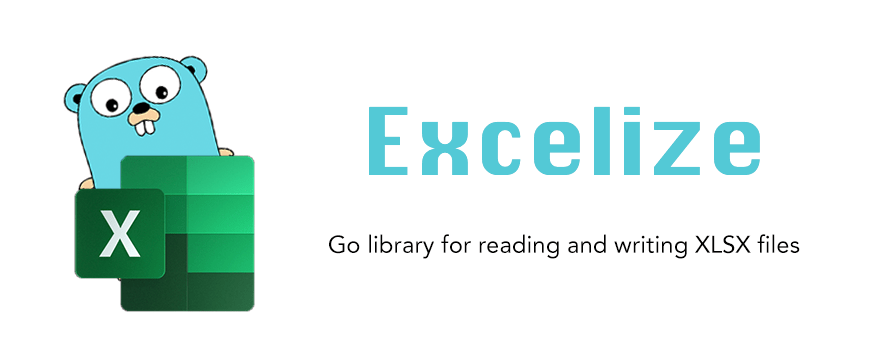﻿ Go 语言 Excel 类库 Excelize 2.0.0 版本发布-Linuxeden开源社区

# Go 语言 Excel 类库 Excelize 2.0.0 版本发布Excelize 是 Go 语言编写的用于操作 Office Excel 文档类库，基于 ECMA-376 Office OpenXML 标准。可以使用它来读取、写入由 Microsoft Excel™ 2007 及以上版本创建的 XLSX 文档。相比较其他的开源类库，Excelize 支持写入原本带有图片(表)、透视表和切片器等复杂样式的文档，还支持向 Excel 文档中插入图片与图表，并且在保存后不会丢失文档原有样式，可以应用于各类报表系统中。

2019年4月23日， 社区正式发布了 2.0.0 版本，该版本包含了很多新功能、错误修复和性能优化。下面是有关该版本更新内容的摘要，完整的更改列表可查看 change log.

## Release Notes

#### API 更改

ToALphaString × × ×
TitleToNumber × × ×
SplitCellName × × ×
JoinCellName × × ×
ColumnNameToNumber × × ×
ColumnNumberToName × × ×
CellNameToCoordinates × × ×
CoordinatesToCellName × × ×
DuplicateRowTo × × ×
SetCellFloat × × ×
SetCellStyle × × ×
InsertCol × × ×
RemoveCol × × ×
RemoveRow × ×
InsertRow × ×
DuplicateRow × × ×
SetRowHeight × × ×
GetRowHeight × × ×
GetCellValue × × ×
GetCellFormula × × ×
SetCellInt × × ×
SetCellBool × × ×
SetCellStr × × ×
SetCellDefault × × ×
GetCellStyle × × ×
SetCellValue × × ×
MergeCell × × ×
SetSheetRow × × ×
SetRowVisible × ×
GetRowVisible × ×
SetRowOutlineLevel × ×
GetRowOutlineLevel × ×
GetRows × × ×
Columns × × ×
SearchSheet × × ×
GetPicture × × ×
AutoFilter × × ×
GetColVisible × × ×
SetColVisible × × ×
GetColOutlineLevel × × ×
SetColOutlineLevel × × ×
SetColWidth × × ×
GetColWidth × × ×
GetMergeCells × × ×
ProtectSheet × × ×
UnprotectSheet × × ×
SetSheetVisible × × ×
prepareCell × × ×
setDefaultTimeStyle × × ×
timeToExcelTime × × ×
getTotalRowsCols × × ×
checkRow × × ×
copySheet × × ×

* 从版本 v2.0.0 开始，所有行操作方法都使用从 `1` 开始的 Excel 行编号，早期版本中某些方法中的基于 `0` 的行编号将不再使用，参考相关 issue #349

#### 问题修复

• 修复部分情况下，在样式格式中未指定单元格颜色填充时，创建了空白填充的问题
• 修复当工作表数据验证公式超过 `225` 个字符时，文件打开失败的问题，解决了相关 issue #339
• 修复部分情况下，删除带有公式的单元格会导致文件损坏的问题，解决了相关 issue #346
• 修复通过获取批注函数 `GetComment()`，从包含多个工作表的工作簿获取批注时，返回了错误的工作表名称的问题，解决了相关 issue #345
• 修复获取工作表全部单元格函数 `GetRows()` 返回多余的列的问题
• 修复部分情况下删除包含公式的工作表引起文档损坏的问题

#### 性能提升

• 单元格赋值系列函数、添加批注、图片、图表、超链接和形状函数的性能，均获得了大幅提升
• 新增重复图片检查，将用添加引用代替添加重复图片，解决了相关 issue #359

#### 其他

• 文档内容更新与修正
• 完善单元测试，增加 go1.12 版本下的单元测试